Gene Expression Data Analysis Suite (GEDAS)

Distance measures

Distances are measured using distance functions, which follow triangle inequality i.e.

d (,£ d (,)  + d (,)

In general language is inequality means the shortest distance between two points as a straight line.   None of the correlation coefficient based distance functions satisfy the triangle inequality and hence are known as semi metric.

In order to cluster gene expression data into groups with similar genes or microarrays, it is necessary to define what exactly is meant by gene expression being identical or gene being similar.  The GEDAS software as described earlier has been as a modified version of the Cluster 3.0 software by de Hoon et al (2004) and includes a number of new clustering/classification algorithms as well as visualization features.

Computation of distance measure (sometimes also referred to as similarity or dissimilarity measure) is one such new feature.  From the existing 8 measures, the number of distance measures has been enhanced to 19 in GEDAS.  The distance measures implemented in the GEDAS software covered the following:

 Sl. No. Distance or similarity measure Expression, Remarks 1. Euclidean distanceIt is a true metric, as it satisfies the triangle inequality, and is the most widely used distance measure of all available.  In this formula, the expression data xi and yi are subtracted directly from each other. We should therefore make sure that the expression data are properly normalized when using the Euclidean distance, for example by converting the measured gene expression levels to log-ratios.   Unlike the correlation-based distance functions, the Euclidean distance takes the magnitude of the expression data into account. It, therefore, preserves more information about the data and may be preferable.  De Hoon et al. (2002) used the Euclidean distance for k-means clustering. 2. Normalized Euclidean distance d =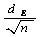where dE is the Euclidean distance 3. Harmonically summed Euclidean distance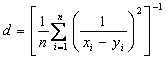It is a variation of the Euclidean distance, where the terms for the different dimensions are summed inversely (similar to the harmonic mean), and is more robust against outliers compared to the Euclidean distance. Note that it is not a metric as it is not based on triangle inequality. For example, consider= (1, 0);= (0, 1);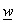= (1, 1). This yields d (,) = 1 while d(,) + d (,) = 0 4. City-block (or Manhattan) distance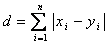Alternatively known as the Manhattan distance or taxi cab distance, it is closely related to the Euclidean distance. Whereas the Euclidean distance corresponds to the length of the shortest path between two points, the city-block distance is the sum of distances along each dimension.   This is equal to the distance a traveler would have to walk between two points in a city. The city-block distance is a metric, as it satisfies the triangle inequality. As for the Euclidean distance, the expression data are subtracted directly from each other, and therefore should be made sure that they are properly normalized. 5. Normalized City-block distanceor  d  =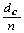where dC is the city block distance 6. Canberra distance d =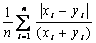, Result always falls in the range [0, 1] 7. Bray-Curtis distance d =, Result always falls in the range [0, 1] 8. Maximum coordinate difference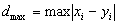Computes maximum absolute difference along a coordinate 9. Minimum coordinate differenceComputes the minimum absolute difference along a coordinate 10. Dot product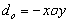, whereThis is the dissimilarity version of the dot product measure 11. Pearson’s correlation coefficient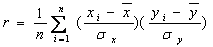The Pearson distance is then defined as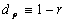In which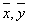are the sample mean of x and y respectively, and σx , σy are the sample standard deviation of x and y. It is a measure for how well a straight line can be fitted to a scatter plot of x and y. If all the points in the scatter plot lie on a straight line, the Pearson correlation coefficient is either +1 or -1, depending on whether the slope of line is positive or negative. If it is equal to zero, there is no correlation between x and y.  As the Pearson correlation coefficient fall between [-1, 1], the Pearson distance lies between [0, 2]. 12. Absolute Pearson’s correlation By taking the absolute value of the Pearson correlation, a number between [0, 1] is obtained. If the absolute value is 1, all the points in the scatter plot lie on a straight line with either a positive or a negative slope. If the absolute value is equal to 0, there is no correlation between x and y. The distance is defined as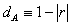where r is the Pearson correlation coefficient. As the absolute value of the Pearson correlation coefficient falls in the range [0, 1], the corresponding distance falls between [0, 1] as well.  In the context of gene expression experiments, the absolute correlation is equal to 1 if the gene expression data of two genes/microarrays have a shape that is either exactly the same or exactly opposite. Therefore, absolute correlation coefficient should be used with care. 13. Uncentered Pearson’s correlationwhereand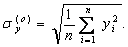The distance corresponding to the uncentered correlation coefficient is defined as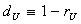where ru is the uncentered correlation.   This is the same as for regular Pearson correlation coefficient, except that sample meansare set equal to 0.  The uncentered correlation may be appropriate if there is a zero reference state.  For instance, in the case of gene expression data given in terms of log-ratios, a log-ratio equal to 0 corresponds to green and red signal being equal, which means that the experimental manipulation did not affect the gene expression.  As the uncentered correlation coefficient lies in the range [-1, 1], the corresponding distance falls between [0, 2]. 14. Absolute uncentered Pearson’s correlation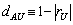where ru is uncentered correlation coefficient. As the absolute value of ru falls between [0, 1], the corresponding distance also falls between [0, 1]. 15. Pearson’s linear dissimilarity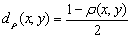, where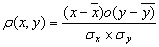This is the dissimilarity version of the Pearson linear correlation r between two vectors.  Dp(i, j) Î [0, 1] with 0 indicating perfect similarity and 1 indicating maximum dissimilarity. Notice that r(I, j) is a type of normalized dot product. 16. Pearson’s absolute value dissimilarity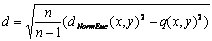where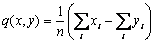dNormEuc is the normalized Euclidean distance 17. Spearman’s rank correlation As in the case of the Pearson correlation, a distance measure corresponding to the Spearman rank correlation can be defined aswhere rs is the Spearman rank correlation.   The Spearman rank correlation is an example of a non-parametric similarity measure. It is useful because it is more robust against outliers than the Pearson correlation.  To calculate the Spearman rank correlation, each data value is replaced by their rank if the data in each vector is ordered by their value. Then the Pearson correlation between the two rank vectors instead of the data vectors is calculated.  Weights cannot be suitably applied to the data if the Spearman rank correlation is used, especially since the weights are not necessarily integers. 18. Kendall’s t distance A distance measure corresponding to Kendall’s τ can be defined asKendall’s τ is another example of a non-parametric similarity measure. It is similar to the Spearman rank correlation, but instead of the rank those only, the relative ranks are used to calculate τ. As in the case of the Spearman rank correlation, the weights are ignored in the calculation.  As Kendall’s τ is defined such that it will lie between [-1, 1], the corresponding distance will in the range [0, 2]. 19. Cosine distance d =Table: Distance measures implemented in GEDAS